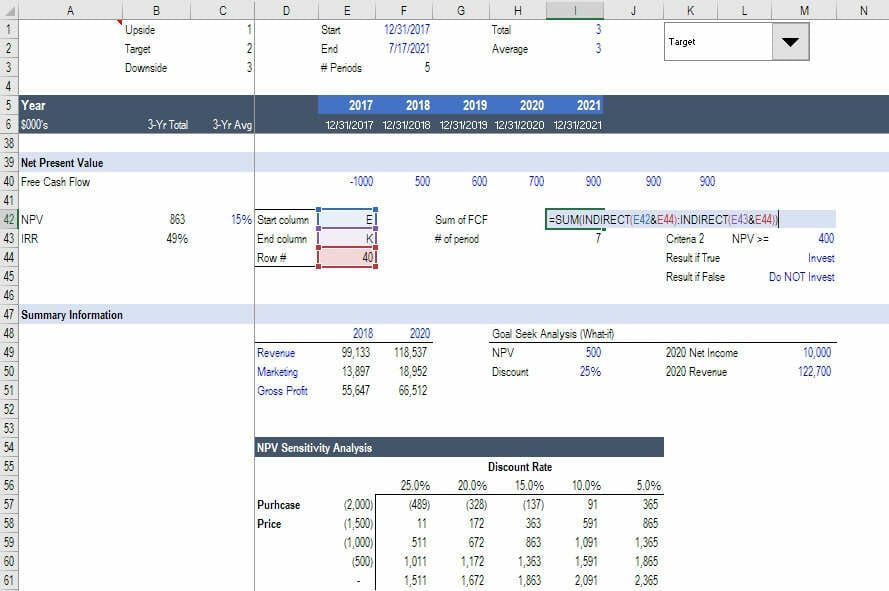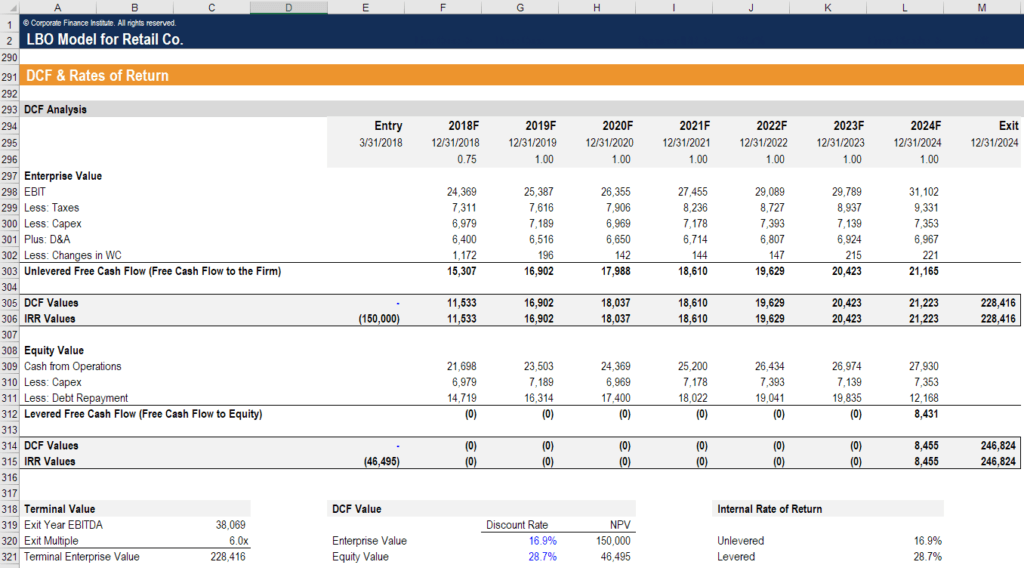# Financial Modeling Formulas

## What are Financial Modeling Formulas?

The most common financial modeling formulas are: =SUM(), =AVERAGE(), =IF(), =INDEX(), =MATCH(), =CHOOSE(), =OFFSET(), =SUMPRODUCT(), =CELL(), XNPV(), and XIRR(). In addition to the formulas, there are various functions that are also important such as Data Validation, What-If Analysis, Scenario Manager, Grouping, Freezing Panes, Trading Dependents / Precedents, and general formatting best practices.CFI is the official global provider of financial modeling and valuation analyst FMVA Designation. CFI’s mission is to help anyone become a world-class financial analyst and has a wide range of resources to help you along the way.

In order to become a great financial analyst, below are some additional questions and answers for you to explore further:

• What is are the types of financial models?
• What is sensitivity analysis?
• What is bookkeeping?
• What are the most common valuation methods?

### Example Excel Model

Below is a screenshot from one of CFI’s online analyst training and certification courses, offered 100% online.

To learn How to Build an Excel Model step-by-step, click on the image below.